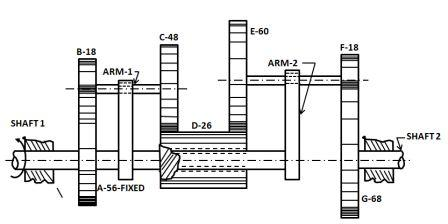# A compound epicyclic train. Shaft 1 is driven at 300 rpm CCW and gear A is fixed to the ground....

## Question:

A compound epicyclic train. Shaft 1 is driven at 300 rpm CCW and gear A is fixed to the ground. The tooth numbers are indicated in the figure. Determine the speed and direction of shaft 2.## Epicyclic Gear train:

An epicyclic gear train is a compound case which consists of several gears arranged in a specific style in order to form a system of gear which is used to transmit power from one shaft to another with a minimum size of the gear box.

Become a Study.com member to unlock this answer! Create your account

{eq}\textbf {Given:} {/eq}

• Number of teeth on the gear A, {eq}T_A=56 {/eq}.
• Number of teeth on the gear B, {eq}T_B=18 {/eq}.
• Number of teeth on...Practice Applying Rotational Motion Formulas

from

Chapter 17 / Lesson 15
2.4K

This lesson introduces the reader to rotational motion and gives examples of solving rotational motion problems such as calculating the angular velocity of an object, the rotational motion of a cylinder, and the angular kinetic energy of an object.